Technical Information Site of Power Supply Design

• TECH INFO
• What capacitor and inductor are the best for a switching power supply? - Inductor- : Matters for Study in Power Supply Circuits

What capacitor and inductor are the best for a switching power supply?

# Matters for Study in Power Supply Circuits

## Inductor －Part 3－

Keyword DC bias currentTemperature rise currentSaturation current

－Up till this point, we have heard about inductor specifications and their characteristics and features. From here on, could you please explain the kinds of effects these characteristics have on power supply circuits.

Certainly. Let's begin by explaining the relationship of the output current of a step-down converter to the DC bias current and the temperature rise current, which were described earlier as important inductor characteristics. To start with, please look at the graphic below.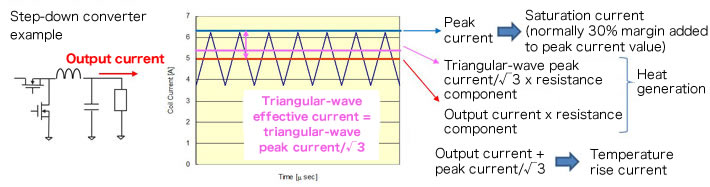The DC bias current is also called the saturation current. In the waveform diagram, the inductor peak current, indicated by a blue line, corresponds to this current. We have already explained a few times previously that, when the peak current exceeds the maximum value for the DC bias current, magnetic saturation of the inductor occurs, and the inductance decreases. When the inductor reaches saturation, the peak current of the inductor becomes abnormally large, resulting in reduced efficiency and abnormal operation, and in the worst case, culminating in destruction of the power supply IC.

The temperature rise current can be restated as the allowable amount of heat generation resulting from the current flowing in the inductor and the resistance component. In the waveform diagram, the total of the DC current in the output, indicated by the red line, and the triangular-wave effective current, shown in pink, corresponds to the temperature rise current. This value multiplied by the resistance component of the inductor becomes the power loss, which generates heat. It should also be noted that the triangular-wave effective current is 1/√3 of the peak current; this part becomes the AC loss. If a current larger than the temperature rise current flows, heat generation becomes prominent, and there is the possibility of a drop in reliability not only of the inductor itself, but of other nearby components as well. Moreover, if heat generation is larger than what can be tolerated, wire insulation faults may occur, possibly leading to burnout.

－Could you explain in a little more detail the phenomena that occur when the peak current has exceeded the DC bias current?

There is actual waveform data, so please take a look.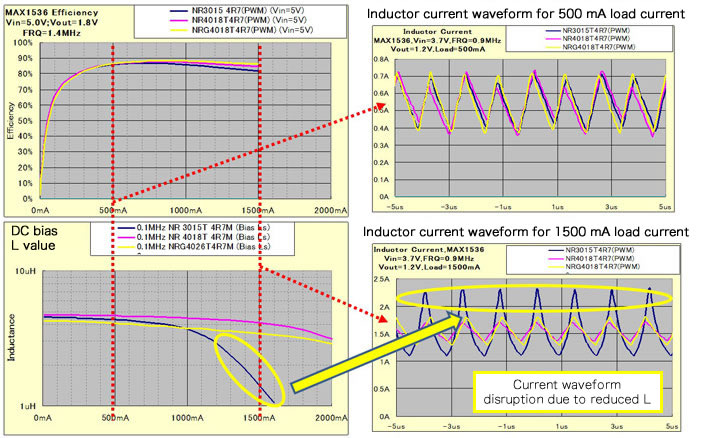This is data for step-down converters using three types of inductors with different characteristics. The output current versus efficiency is shown on the upper left; there is not much difference for a load of 500 mA, but at 1500 mA we see that the efficiency of the inductor represented by the blue line is lower than that of the others.

The waveform on the lower left is the change in inductance with the output current; the inductance of the inductor shown in blue falls suddenly when the load exceeds 1000 mA. In other words, the inductor enters a saturated state.

The right side shows inductor current waveforms. The upper right is for a load of 500 mA, and in this state, there is no particular difference in efficiency; the waveforms are normal, with no notable differences.

The waveform on the lower right is for a load of 1500 mA; the waveform of the inductor shown in blue is considerably disordered, and the peak current is increased. This is because saturation has caused the inductance to drop, so that the peak current is increased, consequently the inductance falls even more, and more current flows, in a so-called runaway phenomenon.

－This means that if an increase in load current makes the efficiency fall below what was expected, one thing to do is to check the waveform of the inductor current.。

I think this is essentially a matter that must be checked in the stage of evaluation of a DC/DC converter. If such an abnormal waveform were observed, or if the peak waveform greatly exceeds the calculated value, one should suspect inductor saturation. I will also note that saturation occurs suddenly in the drum sleeve-type inductors described previously, so that such devices have a propensity for runaway behavior. In resin type devices, saturation is more gradual, so that runway occurs somewhat less readily.

－I see. Next, in relation to the temperature rise current, could you please describe in some detail the inductor losses in particular?

Previously we explained that the parameters deserving study are the DC current in the output, the effective AC current of the inductor, and the resistance component of the inductor. This basically remains the case, and we will consider both the AC losses due to the AC current and the DC losses due to the DC current; however, it is necessary to consider that the DC resistance Rdc and the AC resistance Rac of the inductor are not the same, and that Rac changes depending on the frequency.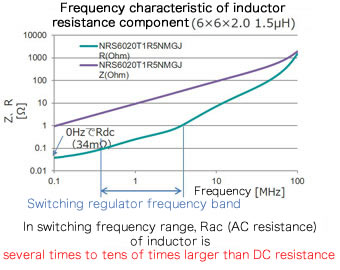This graph shows the relation between resistance, impedance, and frequency for a certain inductor. The green line is the resistance, and the value of Rdc is at zero frequency. On the other hand, as the frequency rises, Rac increases, and in the range from hundreds of kHz to several MHz, which is the switching frequency range of switching regulators in general, Rac is from several times to several tens of times the value of Rdc.

－In other words, AC losses are large even for small currents. And they are even higher when the switching frequency is high.

That's right. And the bulk of the losses changes depending on the size of the load current. This is probably easier to understand by considering the following graphic.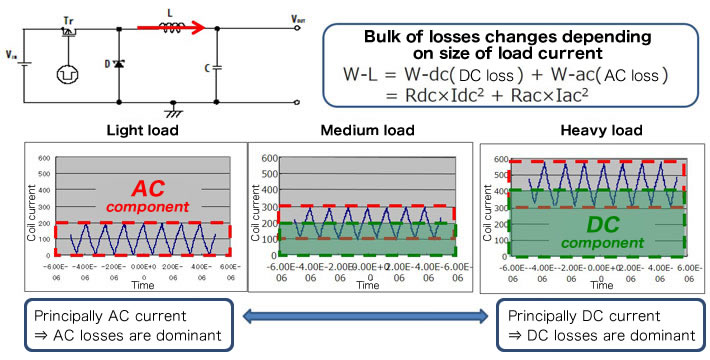As indicated in the equation above, the power loss of an inductor is equal to the DC power loss plus the AC power loss. If we first consider a light loading state, the output current is small, so that DC losses are small, and nearly all the current is the AC component, so that AC losses are dominant. In a state of heavy loading, that is, when the output current is large, it is the DC current that is dominant.

And regarding another thing, please look at this data as well. This compares the load-efficiency characteristics of inductors with the same inductance, but with different structures, sizes, and allowable currents when used in the same DC/DC converter circuit, and also shows the inductor Rac frequency characteristic of each.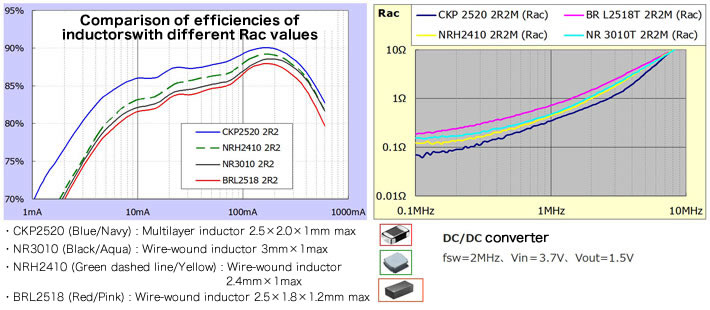In the large-load region, the DC losses due to the inductor Rdc are dominant. Under light loading, AC losses due to Rac are dominant. What I would like you to focus on here is that, although there is some variation in both the Rdc and the Rac depending on the inductor type, the variation in Rac is particularly large.

From the efficiency graph, it can be seen that the differences in efficiency are larger under light loading, and so the Rac differences are large. And from the Rac graph, it is clear that there is considerable variation in Rac itself. The vertical axis is plotted on a log scale, and so there is a difference of several-fold between the values for the inductors represented by the pink and the blue lines.

－I see that AC losses are dominant under light loads, and that there is considerable variation depending on the inductor type, but does this imply some kind of problem?

For example, in equipment that tends to be in a wait state for a long time, such as smartphones, much of the time of operation is in the standby state, and there is only a very small load current, which is a DC current. Thus in this state AC losses dominate, and although the current is very small, losses can be comparatively large. What then would happen if an inductor with a high Rac were used in such equipment.

－The wait time would be shortened, that is to say, the battery durability might be worsened.

Yes. the power source is a battery, and so one wants to reduce the losses during standby. If the designer were not aware of such characteristics of inductors and selected an inductor with a high Rac, the desired operating time might not be achieved in portable equipment driven by a battery. In battery-driven equipment with long periods in standby, it is important that the Rac value be low.

－So rather than merely focusing on heat generation due to inductor losses, it is important that the causes of losses and loss characteristics be understood when choosing an inductor. By the way, in the inductor specifications explained at the beginning, the Rdc was listed, but I don't think Rac was included.

Rac almost never appears in specifications tables, and is probably not described very often on the product websites either. When Rac information is necessary, it is a good idea to ask the manufacturer.

## What capacitor and inductor are the best for a switching power supply? Inductor – Part 2